Next: 8.6 Integrals and Area Up: 8. Integrable Functions Previous: 8.4 The Ruler Function   Index

# 8.5 Change of Scale

8.42   Definition (Stretch of a function.) Let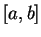be an interval in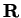, let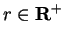, and let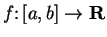. We define a new function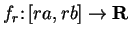by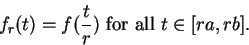If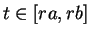, then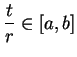, so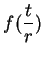is defined.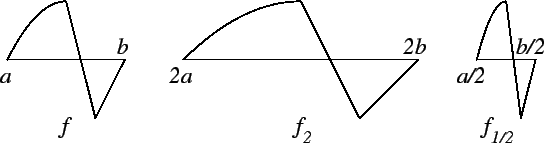The graph of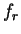is obtained by stretching the graph of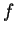by a factor of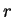in the horizontal direction, and leaving it unstretched in the vertical direction. (If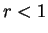the stretch is actually a shrink.) I will callthe stretch ofby.

8.43   Theorem (Change of scale for integrals.) Letbe an interval inand let. Letand letbe the stretch ofby. Ifis integrable onthenis integrable on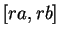and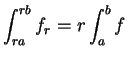, i.e.,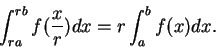(8.44)

Proof: Supposeis integrable on. Let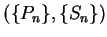be an arbitrary partition-sample sequence for. If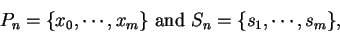let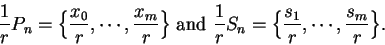Then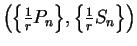is a partition-sample sequence for, so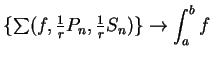. Now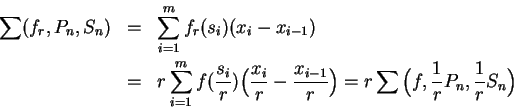so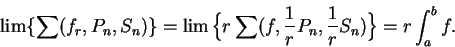This shows thatis integrable on, and.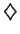Remark: The notationis not a standard notation for the stretch of a function, and I will not use this notation in the future. I will usually use the change of scale theorem in the form of equation (8.44), or in the equivalent form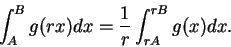(8.45)

8.46   Exercise. A Explain why formula (8.45) is equivalent to formula (8.44).

8.47   Example. We define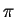to be the area of the unit circle. Since the unit circle is carried to itself by reflections about the horizontal and vertical axes, we have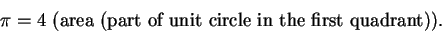Since points in the unit circle satisfy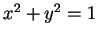or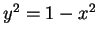, we get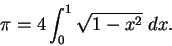We will use this result to calculate the area of a circle of radius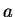. The points on the circle with radiusand center 0 satisfy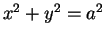, and by the same symmetry arguments we just gave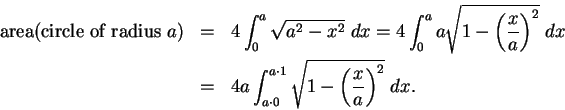By the change of scale theorem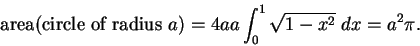The formulas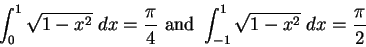or more generally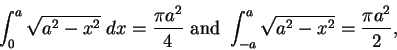are worth remembering. Actually, these are cases of a formula you already know, since they say that the area of a circle of radiusis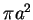.

8.48   Exercise. A Let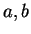be positive numbers and let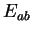be the set of points inside the ellipse whose equation is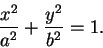Calculate the area of.

8.49   Exercise. The figure shows the graph of a function.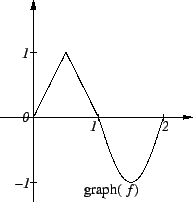Let functions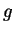,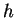,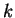,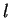, and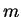be defined by
a)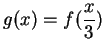.
b)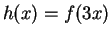.
c)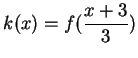.
d)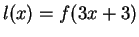.
e)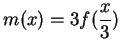.
Sketch the graphs of,,,, andon different axes. Use the same scale for all of the graphs, and use the same scale on the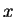-axis and the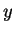-axis,

8.50   Exercise. A The value of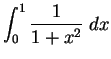is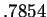(approximately). Use this fact to calculate approximate values for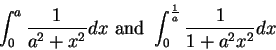where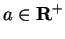. Find numerical values for both of these integrals when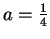.Next: 8.6 Integrals and Area Up: 8. Integrable Functions Previous: 8.4 The Ruler Function   Index
Ray Mayer 2007-09-07